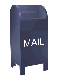This is an edited verson of a question I posted to comp.lang.c++ in July 2003. John Harrison was kind enough to answer this question, which helped me develop version 3.0 of my reference counted String class. Apparently John is the author of a reference counted String class for the Dinkumware STL library.

The answer to the question posed here is that a proxy class must be used to properly implement copy-on-write. This proxy class is used in my reference counted String and the templates that it is based on.

### How do you overload operator [] to implement copy-on-write

Say I want to develop a class that supports the overloaded [] operator and reads and writes the "int" type. I thought that the way this was done was:

```    class MyClass
{
//...
// in theory, the RHS operator
const int operator[](const int i ) const;
// in theory, the LHS operator
int& operator[](const int i );
//...
}
```

Here RHS stands for right-hand-side, or an r-value and LHS stands for left-hand-side, or an l-value.

```    MyClass foo;

int i = foo[j]; // RHS reference  NOT!
foo[j] = i;     // LHS reference
```

Much to my surprise, the first statement "i = foo[j];" seems to invoke the overloaded operator I've labeled LHS. I tried this with Microsoft's Visual C++ 6.0 compiler, I think upgraded with at least service pack 5 (version 12.00.8804) and the GNU 2.95.2 g++ compiler for Intel on freeBSD. Both compilers got the same results.

To put things in more concrete form, I've included a complete test code below:

```#include <stdio.h>

{
private:
int *pArray;

public:
{
pArray = new int[ size ];
}

{
delete [] pArray;
}

// in theory, the RHS operator
const int operator[](const int i ) const
{
printf("RHS a[%2d]\n", i );
return pArray[i];
}

// in theory, the LHS operator
int& operator[](const int i )
{
printf("LHS a[%2d]\n", i );
return pArray[i];
}

int
main()
{
const int len = 4;
int b[len];

int i;

printf("initializing array...\n");
for (i = 0; i < len; i++) {
a[i] = i + 1;
}

printf("reading values from array in an 'if' statement...\n");
for (i = 0; i < len; i++) {
if (a[i] != i+1) {
break;
}
}

printf("reading values from an array in an assignment...\n");
for (i = 0; i < len; i++) {
b[i] = a[i];
}

printf("expression...\n");
int j = a + a;
return 0;
}
```

When I compile and execute this code I get

```initializing array...
LHS a[ 0]
LHS a[ 1]
LHS a[ 2]
LHS a[ 3]
reading values from array in an 'if' statement...
LHS a[ 0]
LHS a[ 1]
LHS a[ 2]
LHS a[ 3]
reading values from an array in an assignment...
LHS a[ 0]
LHS a[ 1]
LHS a[ 2]
LHS a[ 3]
expression...
LHS a[ 1]
LHS a[ 2]
```

I expected that the "initialization" would reference the operator [] function I've labeled LHS. However, much to my surprise, the 'if' statement and the value reads also referenced the function labeled LHS. I'm surprised at this, since as far as I can tell, the way I've implemented the overloaded [] operators is pretty much the "text book" approach.

Is there a way to implement this class so that the RHS [] will be called when it seems to be an r-value? For example, the references to a below:

```    if (a[i] != i+1)
b[i] = a[i];
int j = a + a;
```

In this example the difference is not critical, since the code gets the expected results. However, proper invokation of the RHS and LHS operators is important in the case of reference counted objects, with copy-on-write semantics, which is the appliction that originally motivated this question.

I'm working on version 3.0 of a reference counted String class, which can be found here: http://www.bearcave.com/software/string/index.html. Version 2.0 of this class suffers from a bug caused by the behavior of the [] operator described above. In particular, it is making copies on read.

I have noted Stroustrup's solution using the Cref class (from 11.12 of The C++ Programming Language, Third Edition).

Stroustrup's Cref class served as the model for the ValRef class which is local to the RCBase template in version 3.0 of the String class. The ValRef class is returned by the operator [] function. This class implements the operator = which is only invoked when the ValRef object is referenced as an l-value.

Ian Kaplan, September 2003

back to String Container Class